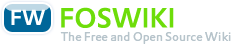# Course Mathematics 1 Kunz

Responsible: Prof. Dr. Dietmar Kunz

## Course

### Meets requirements of following modules(MID)

• in active programs

### Course Organization

Version
created 2011-11-09
VID 1
valid from WS 2012/13
valid to
Course identifiers
Long name Mathematics 1 Kunz
CID F07_MA1_Kunz
CEID (exam identifier)

Contact hours per week (SWS)
Lecture 5
Exercise (unsplit)
Exercise (split) 2
Lab
Project
Seminar
Tutorial(voluntary) 2
Total contact hours
Lecture 75
Exercise (unsplit)
Exercise (split) 30
Lab
Project
Seminar
Tutorial (voluntary) 30
Max. capacity
Exercise (unsplit)
Exercise (split) 40
Lab
Project
Seminar

Total effort (hours): 300

• German

#### Prerequisites

• tangible school knowledge

• Fetzer/Frankel: Mathematik
• Papula: Mathematik

#### Instructors

• Prof. Dr. Dietmar Kunz
• Prof. Dr. Stefan Grünvogel

• tba

Mathematics 1

### Assessment

Type
wE if directly following course: 30% midterm exam, 70% concluding written exam
wE if not directly following course: 100%

Total effort [hours]
wE 10

Frequency: 2/year

## Course components

### Lecture/Exercise

#### Objectives

##### Contents
• basics
• statements
• sets
• natural numbers
• factorial
• binomial theorem
• mathematical induction
• real numbers
• modulus
• (in)equalities
• functions
• monotonous
• bounded
• symmetry
• elementary functions
• algebraic functions
• division of polynomials
• Horner's method
• partial fraction decomposition
• transcendental functions
• trigonometric functions
• exp and log
• geometry
• coordinate systems
• analysis
• convergence, divergence
• sequences
• functions
• continuity
• complex numbers
• linear algebra
• vectors
• linear (in)dependence
• scalar product
• angle between vectors
• norm
• orthogonal decomposition
• cross product
• iterated cross products
• angle between planes
• spherical angle, spherical triangles
• matrices
• linear equation systems
• Gaussian elimination
• inversion
• determinants
• orthogonal matrices
• homogeneous coordinates
• eigenvalues, -vectors

##### Acquired Skills
• solve exercises concerning
• basics
• statements
• sets
• natural numbers
• factorial
• binomial theorem
• mathematical induction
• real numbers
• modulus
• (in)equalities
• functions
• monotonous
• bounded
• symmetry
• elementary functions
• algebraic functions
• division of polynomials
• Horner's method
• partial fraction decomposition
• transcendental functions
• trigonometric functions
• exp and log
• geometry
• coordinate systems
• analysis
• convergence, divergence
• sequences
• functions
• continuity
• complex numbers
• linear algebra
• vectors
• linear (in)dependence
• scalar product
• angle between vectors
• norm
• orthogonal decomposition
• cross product
• iterated cross products
• angle between planes
• spherical angle, spherical triangles
• matrices
• linear equation systems
• Gaussian elimination
• inversion
• determinants
• orthogonal matrices
• homogeneous coordinates
• eigenvalues, -vectors

##### Operational Competences
• solve application-related problems

Type
wE written midterm exam about 8 weeks after start
fPS exrcises during lecture and as home workDeutsch English